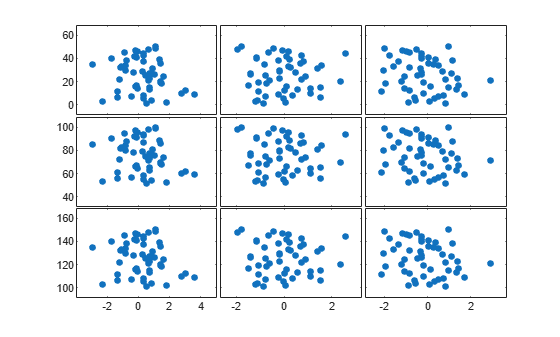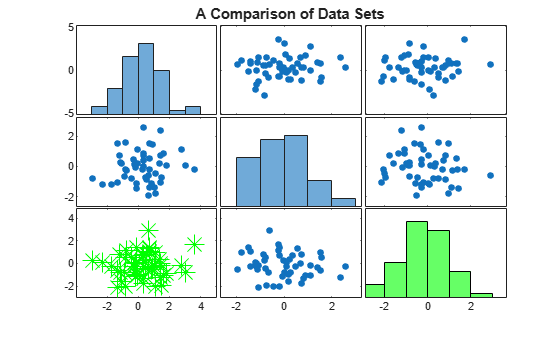# plotmatrix

Scatter plot matrix

## Syntax

``plotmatrix(X,Y)``
``plotmatrix(X)``
``plotmatrix(___,LineSpec)``
``plotmatrix(ax,___)``
``````[S,AX,BigAx,H,HAx] = plotmatrix(___)``````

## Description

example

````plotmatrix(X,Y)` creates a matrix of subaxes containing scatter plots of the columns of `X` against the columns of `Y`. If `X` is p-by-n and `Y` is p-by-m, then `plotmatrix` produces an n-by-m matrix of subaxes.```

example

````plotmatrix(X)` is the same as `plotmatrix(X,X)` except that the subaxes along the diagonal are replaced with histogram plots of the data in the corresponding column of `X`. For example, the subaxes along the diagonal in the ith column is replaced by `histogram(X(:,i))`.```

example

````plotmatrix(___,LineSpec)` specifies the line style, marker symbol, and color for the scatter plots. The option `LineSpec` can be preceded by any of the input argument combinations in the previous syntaxes.```

example

````plotmatrix(ax,___)` plots into the specified target axes, where the target axes is an invisible frame for the subaxes.```

example

``````[S,AX,BigAx,H,HAx] = plotmatrix(___)``` returns the graphic objects created as follows:`S` – Chart line objects for the scatter plots`AX` – Axes objects for each subaxes`BigAx` – Axes object for big axes that frames the subaxes`H` – Histogram objects for the histogram plots`HAx` – Axes objects for the invisible histogram axes`BigAx` is left as the current axes (`gca`) so that a subsequent `title`, `xlabel`, or `ylabel` command centers text with respect to the big axes.```

## Examples

collapse all

Create `X` as a matrix of random data and `Y` as a matrix of integer values. Then, create a scatter plot matrix of the columns of `X` against the columns of `Y`.

```X = randn(50,3); Y = reshape(1:150,50,3); plotmatrix(X,Y)```The subplot in the ith row, jth column of the figure is a scatter plot of the ith column of `Y` against the jth column of `X`.

Create a scatter plot matrix of random data. The subplot in the ith row, jth column of the matrix is a scatter plot of the ith column of `X` against the jth column of `X`. Along the diagonal are histogram plots of each column of `X`.

```X = randn(50,3); plotmatrix(X)```Create a scatter plot matrix of random data. Specify the marker type and the color for the scatter plots.

```X = randn(50,3); plotmatrix(X,'*r')```The `LineSpec` option sets properties for the scatter plots. To set properties for the histogram plots, return the histogram objects.

Create a scatter plot matrix of random data.

```rng default X = randn(50,3); [S,AX,BigAx,H,HAx] = plotmatrix(X);```To set properties for the scatter plots, use `S`. To set properties for the histograms, use `H`. To set axes properties, use `AX`, `BigAx`, and `HAx`. Use dot notation to set properties.

Set the color and marker type for the scatter plot in the lower left corner of the figure. Set the color for the histogram plot in the lower right corner. Use the `title` command to title the figure.

```S(3).Color = 'g'; S(3).Marker = '*'; H(3).EdgeColor = 'k'; H(3).FaceColor = 'g'; title(BigAx,'A Comparison of Data Sets')```## Input Arguments

collapse all

Data to display, specified as a matrix.

Data Types: `single` | `double` | `int8` | `int16` | `int32` | `int64` | `uint8` | `uint16` | `uint32` | `uint64` | `logical`

Data to plot against `X`, specified as a matrix.

Data Types: `single` | `double` | `int8` | `int16` | `int32` | `int64` | `uint8` | `uint16` | `uint32` | `uint64` | `logical`

Line style, marker, and color, specified as a character vector or string containing symbols. The symbols can appear in any order. You do not need to specify all three characteristics (line style, marker, and color). For example, if you omit the line style and specify the marker, then the plot shows only the marker and no line.

Example: `'--or'` is a red dashed line with circle markers

Line StyleDescription
`-`Solid line
`--`Dashed line
`:`Dotted line
`-.`Dash-dot line
MarkerDescription
`'o'`Circle
`'+'`Plus sign
`'*'`Asterisk
`'.'`Point
`'x'`Cross
`'_'`Horizontal line
`'|'`Vertical line
`'s'`Square
`'d'`Diamond
`'^'`Upward-pointing triangle
`'v'`Downward-pointing triangle
`'>'`Right-pointing triangle
`'<'`Left-pointing triangle
`'p'`Pentagram
`'h'`Hexagram
ColorDescription

`y`

yellow

`m`

magenta

`c`

cyan

`r`

red

`g`

green

`b`

blue

`w`

white

`k`

black

Target axes that frames all the subaxes, specified as an `Axes` object. If you do not specify this argument, then `plotmatrix` uses the current axes.

## Output Arguments

collapse all

Chart line objects for the scatter plots, returned as a matrix. These are unique identifiers, which you can use to query and modify the properties of a specific scatter plot.

Axes objects for the subaxes, returned as a matrix. These are unique identifiers, which you can use to query and modify the properties of a specific subaxes.

Axes object for big axes, returned as a scalar. This is a unique identifier, which you can use to query and modify properties of the big axes.

Histogram objects, returned as a vector or `[]`. These are unique identifiers, which you can use to query and modify the properties of a specific histogram object. If no histogram plots are created, then `H` is returned as empty brackets.

Note

Starting in R2015b, `H` is a vector of histogram objects, In previous releases, it was a vector of patch objects.

Axes objects for invisible histogram axes, returned as a vector or `[]`. These are unique identifiers, which you can use to query and modify the properties of a specific axes. If no histogram plots are created, then `HAx` is returned as empty brackets.